# 4.3 Mpeg layers 1-3: cosine-modulated filterbanks

 Page 1 / 1
Here the "polyphase quadrature" filterbank used in the MPEG audio standards is described in great detail. It has the following practical features: real-valued sub-band outputs, near-perfect reconstruction, and polyphase implementation; and is based on cancellation of adjacent sub-band interference.
• Though the uniformly modulated filterbank in Figure 4 from "Uniformly-Modulated Filterbanks" was shown to have the fast implementation in Figure 5 from "Uniformly-Modulated Filterbanks" , the sub-band outputs are complex-valued for real-valuedinput, hence inconvenient (at first glance In the structure in Figure 4 from "Uniformly-Modulated Filterbanks" , it would be reasonable to replace the standard DFT with a real-valued DFT (defined inthe notes on transform coding), requiring $\approx N{log}_{2}N$ real-multiplies when N is a power of 2. Though it is not clear to the author why such a structure was notadopted in the MPEG standards, the cosine modulated filterbank derived in this section has equivalent performance and, withits polyphase/DCT implementation, equivalent implementation cost. ) for sub-band coding of real-valued data. In this section we propose a closely related filterbank with thefollowing properties.
1. Real-valued sub-band outputs (assuming real-valued inputs),
2. Near-perfect reconstruction,
3. Polyphase/fast-transform implementation.
This turns out to be the filterbank specified in the MPEG-1 and 2 (layers 1-3) audio compression standards (see IS0/IEC 13818-3).

## Filter design

• Real-valued Sub-band Outputs: Recall the generic filterbank structure of Figure 1 from "Uniformly-Modulated Filterbanks" . For the sub-band outputs to be real-valued (for real-valued input),we require that the impulse responses of $\left\{{H}_{i}\left(z\right)\right\}$ and $\left\{{K}_{i}\left(z\right)\right\}$ are real-valued. We can insure this by allocating the N (symmetric) frequency band pairs shown in [link] . The positive and negative halves of each band pair are centered at ${\omega }_{i}=\frac{\left(2i+1\right)\pi }{2N}$ radians.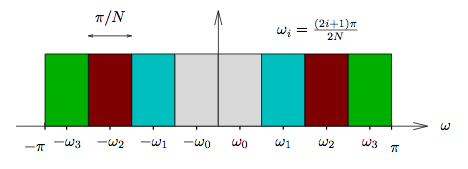Frequency band pairs for the polyphase quadrature filterbank ( N = 4 ). We can consider each filter ${H}_{i}\left(z\right)$ as some combination of symmetric positive-frequency and negative-frequency components
${H}_{i}\left(z\right)={a}_{i}{F}_{i}\left(z\right)+{b}_{i}{G}_{i}\left(z\right)$
as shown in [link] .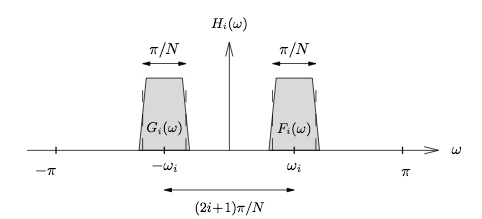Positive- and negative-frequency decomposition of H i ( ω ) . Note K i ( ω ) will have a similar, if not identical, frequency response. When ${b}_{i}={a}_{i}^{*}$ and the pairs $\left\{{F}_{i}\left(z\right),{G}_{i}\left(z\right)\right\}$ are modulated versions of the same prototype filter $H\left(z\right)$ , we can show that ${H}_{i}\left(z\right)$ must be real-valued:
$\begin{array}{ccc}\hfill {H}_{i}\left(z\right)& =& {a}_{i}\underset{{F}_{i}\left(z\right)}{\underbrace{H\left({e}^{-j\pi \frac{2i+1}{2N}}z\right)}}\phantom{\rule{0.222222em}{0ex}}+\phantom{\rule{0.277778em}{0ex}}{a}_{i}^{*}\underset{{G}_{i}\left(z\right)}{\underbrace{H\left({e}^{j\pi \frac{2i+1}{2N}}z\right)}}\hfill \\ & =& {a}_{i}\sum _{n}{h}_{n}{e}^{j\pi \frac{2i+1}{2N}n}{z}^{-n}+{a}_{i}^{*}\sum _{n}{h}_{n}{e}^{-j\pi \frac{2i+1}{2N}n}{z}^{-n}\hfill \\ & =& \mathrm{Re}\left({a}_{i}\right)\sum _{n}{h}_{n}{z}^{-n}\left({e}^{j\pi \frac{2i+1}{2N}n},+,{e}^{-j\pi \frac{2i+1}{2N}n}\right)+j\mathrm{Im}\left({a}_{i}\right)\sum _{n}{h}_{n}{z}^{-n}\left({e}^{j\pi \frac{2i+1}{2N}n},-,{e}^{-j\pi \frac{2i+1}{2N}n}\right)\hfill \\ & =& \mathrm{Re}\left({a}_{i}\right)\sum _{n}{h}_{n}{z}^{-n}·2cos\phantom{\rule{-0.166667em}{0ex}}\left(\pi ,\frac{2i\phantom{\rule{-0.166667em}{0ex}}+\phantom{\rule{-0.166667em}{0ex}}1}{2N},n\right)+j\mathrm{Im}\left({a}_{i}\right)\sum _{n}{h}_{n}{z}^{-n}·2jsin\phantom{\rule{-0.166667em}{0ex}}\left(\pi ,\frac{2i\phantom{\rule{-0.166667em}{0ex}}+\phantom{\rule{-0.166667em}{0ex}}1}{2N},n\right)\hfill \\ & =& 2\sum _{n}\left[\mathrm{Re},\left({a}_{i}\right),\phantom{\rule{0.166667em}{0ex}},cos,\phantom{\rule{-0.166667em}{0ex}},\left(\pi ,\frac{2i\phantom{\rule{-0.166667em}{0ex}}+\phantom{\rule{-0.166667em}{0ex}}1}{2N},n\right),-,\mathrm{Im},\left({a}_{i}\right),\phantom{\rule{0.166667em}{0ex}},sin,\phantom{\rule{-0.166667em}{0ex}},\left(\pi ,\frac{2i\phantom{\rule{-0.166667em}{0ex}}+\phantom{\rule{-0.166667em}{0ex}}1}{2N},n\right)\right]{h}_{n}{z}^{-n}\hfill \end{array}$
• Aliasing Cancellation: Recall again the generic filterbank in Figure 1 from "Uniformly-Modulated Filterbanks" . Here we determine conditions on real-valued $\left\{{H}_{i}\left(z\right)\right\}$ and $\left\{{K}_{i}\left(z\right)\right\}$ which lead to near-perfect reconstruction. It will be insightful to derive an expression for the input to the ${i}^{th}$ reconstruction filter, $\left\{{y}_{i}\left(n\right)\right\}$ . The downsample-upsample-cascade equation Equation 14 from "Fundamentals of Multirate Signal Processing" (fourth equation) implies that
$\begin{array}{ccc}\hfill {Y}_{i}\left(z\right)& =& \frac{1}{N}\sum _{p=0}^{N-1}{X}_{i}\left({e}^{-j\frac{2\pi }{N}p},z\right)\hfill \\ & =& \frac{1}{N}\sum _{p=0}^{N-1}{H}_{i}\left({e}^{-j\frac{2\pi }{N}p},z\right)X\left({e}^{-j\frac{2\pi }{N}p},z\right)\hfill \\ & =& \frac{1}{N}\sum _{p=0}^{N-1}\left[{a}_{i},{F}_{i},\left({e}^{-j\frac{2\pi }{N}p},z\right),+,{a}_{i}^{*},{G}_{i},\left({e}^{-j\frac{2\pi }{N}p},z\right)\right]X\left({e}^{-j\frac{2\pi }{N}p},z\right)\hfill \\ & =& \underset{\text{desired}}{\underbrace{\frac{1}{N}\left[{a}_{i},{F}_{i},\left(z\right),+,{a}_{i}^{*},{G}_{i},\left(z\right)\right]X\left(z\right)}}+\underset{\text{undesired}\phantom{\rule{4.pt}{0ex}}\text{images}}{\underbrace{\frac{1}{N}\sum _{p=1}^{N-1}\left[{a}_{i},{F}_{i},\left({e}^{-j\frac{2\pi }{N}p},z\right),+,{a}_{i}^{*},{G}_{i},\left({e}^{-j\frac{2\pi }{N}p},z\right)\right]X\left({e}^{-j\frac{2\pi }{N}p},z\right)}}.\hfill \end{array}$
Thus the input to the ${i}^{th}$ reconstruction filter is corrupted by unwanted spectral images, and the reconstruction filter's job is theremoval of these images. The reconstruction filter ${K}_{i}\left(z\right)$ will have a bandpass frequency response similar (or identical) to that of ${H}_{i}\left(z\right)$ illustrated in [link] . Due to the practical design considerations, neither ${K}_{i}\left(z\right)$ nor ${H}_{i}\left(z\right)$ will be perfect bandpass filters, but we will assume that the only significant out-of-band energy passed by these filters willoccur in the frequency range just outside of their passbands. (Note the limited “spillover” in [link] .) Under these assumptions, the only undesired images in ${Y}_{i}\left(\omega \right)$ that will not be completely attenuated by ${K}_{i}\left(\omega \right)$ are the images adjacent to ${F}_{i}\left(\omega \right)$ and ${G}_{i}\left(\omega \right)$ . Which indices p in [link] (third equation) are responsible for these adjacent images? [link] (third equation) implies that index $p=\ell$ shifts the frequency response up by $2\pi \ell /N$ radians. Since the passband centers of ${F}_{i}\left(z\right)$ and ${G}_{i}\left(z\right)$ are $\left(2i+1\right)\pi /N$ radians apart, the passband of ${G}_{i}\left({e}^{-j\frac{2\pi }{N}p},z\right)$ will reside directly to the left of the passband of ${F}_{i}\left(z\right)$ when $p=i$ . Similarly, the passband of ${G}_{i}\left({e}^{-j\frac{2\pi }{N}p},z\right)$ will reside directly to the right of the passband of ${F}_{i}\left(z\right)$ when $p=i+1$ . See [link] for an illustration. Using the same reasoning, the passband of ${F}_{i}\left({e}^{-j\frac{2\pi }{N}p},z\right)$ will reside directly to the right of the passband of ${G}_{i}\left(z\right)$ when $p=-i$ and directly to the left when $p=-\left(i+1\right)$ . The only exceptions to this rule occur when $i=0$ , in which case the images to the right of ${G}_{i}\left(z\right)$ and to the left of ${F}_{i}\left(z\right)$ are desired, and when $i=N-1$ , in which case the images to the left of ${G}_{i}\left(z\right)$ and to the right of ${F}_{i}\left(z\right)$ are desired.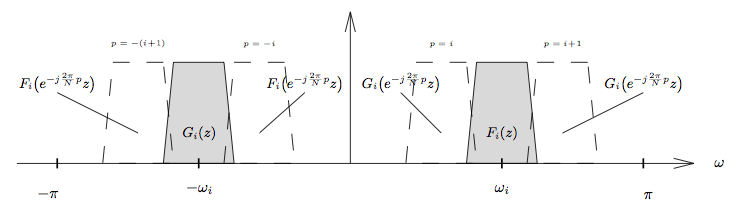Spectral images of Y i ( ω ) not completely attenuated by K i ( ω ) . Based on the arguments above, we can write $\left\{{u}_{i}\left(n\right)\right\}$ , the output of the ${i}^{th}$ reconstruction filter, as follows:
$\begin{array}{ccc}\hfill {U}_{i}\left(z\right)& =& {K}_{i}\left(z\right){Y}_{i}\left(z\right)\hfill \\ & =& \underset{\text{desired}}{\underbrace{\frac{1}{N}{K}_{i}\left(z\right)\left[{a}_{i},{F}_{i},\left(z\right),X,\left(z\right),+,{a}_{i}^{*},{G}_{i},\left(z\right),X,\left(z\right)\right]}}\hfill \\ & & +\underset{\text{aliasing}\phantom{\rule{4.pt}{0ex}}\text{from}\phantom{\rule{4.pt}{0ex}}\text{inner}\phantom{\rule{4.pt}{0ex}}\text{undesired}\phantom{\rule{4.pt}{0ex}}\text{images}\phantom{\rule{4.pt}{0ex}}\text{when}\phantom{\rule{4.pt}{0ex}}1\le i\le N-1}{\underbrace{\frac{1}{N}{K}_{i}\left(z\right)\left[{a}_{i},{F}_{i},\left({e}^{j\frac{2\pi }{N}i},z\right),X,\left({e}^{j\frac{2\pi }{N}i},z\right),+,{a}_{i}^{*},{G}_{i},\left({e}^{-j\frac{2\pi }{N}i},z\right),X,\left({e}^{-j\frac{2\pi }{N}i},z\right)\right]}}\hfill \\ & & +\underset{\text{aliasing}\phantom{\rule{4.pt}{0ex}}\text{from}\phantom{\rule{4.pt}{0ex}}\text{outer}\phantom{\rule{4.pt}{0ex}}\text{undesired}\phantom{\rule{4.pt}{0ex}}\text{images}\phantom{\rule{4.pt}{0ex}}\text{when}\phantom{\rule{4.pt}{0ex}}0\le i\le N-2}{\underbrace{\frac{1}{N}{K}_{i}\left(z\right)\left[{a}_{i},{F}_{i},\left({e}^{j\frac{2\pi }{N}\left(i+1\right)},z\right),X,\left({e}^{j\frac{2\pi }{N}\left(i+1\right)},z\right),+,{a}_{i}^{*},{G}_{i},\left({e}^{-j\frac{2\pi }{N}\left(i+1\right)},z\right),X,\left({e}^{-j\frac{2\pi }{N}\left(i+1\right)},z\right)\right]}}.\hfill \end{array}$
The previous equation shows that ${U}_{i}\left(z\right)$ is corrupted by the portions of the undesired images not completely removed by the reconstructionfilter ${K}_{i}\left(z\right)$ . In the filterbank context, this undesired behavior is referred to asaliasing. But notice that aliasing contributions to the signal $U\left(z\right)={\sum }_{i}{U}_{i}\left(z\right)$ will vanish if the inner aliasing components in ${U}_{i}\left(z\right)$ cancel the outer aliasing components in ${U}_{i-1}\left(z\right)$ . This happens when
$\begin{array}{c}{K}_{i}\left(z\right)\left[{a}_{i},{F}_{i},\left({e}^{j\frac{2\pi }{N}i},z\right),X,\left({e}^{j\frac{2\pi }{N}i},z\right),+,{a}_{i}^{*},{G}_{i},\left({e}^{-j\frac{2\pi }{N}i},z\right),X,\left({e}^{-j\frac{2\pi }{N}i},z\right)\right]\\ \hfill =-{K}_{i-1}\left(z\right)\left[{a}_{i-1},{F}_{i-1},\left({e}^{j\frac{2\pi }{N}i},z\right),X,\left({e}^{j\frac{2\pi }{N}i},z\right),+,{a}_{i-1}^{*},{G}_{i-1},\left({e}^{-j\frac{2\pi }{N}i},z\right),X,\left({e}^{-j\frac{2\pi }{N}i},z\right)\right].\end{array}$
which occurs under satisfaction of the two conditions below.
$\begin{array}{ccc}\hfill {a}_{i}{K}_{i}\left(z\right){F}_{i}\left({e}^{j\frac{2\pi }{N}i},z\right)& =& -{a}_{i-1}{K}_{i-1}\left(z\right){F}_{i-1}\left({e}^{j\frac{2\pi }{N}i},z\right)\hfill \\ \hfill {a}_{i}^{*}{K}_{i}\left(z\right){G}_{i}\left({e}^{-j\frac{2\pi }{N}i},z\right)& =& -{a}_{i-1}^{*}{K}_{i-1}\left(z\right){G}_{i-1}\left({e}^{-j\frac{2\pi }{N}i},z\right).\hfill \end{array}$
We assume from this point on that the real-valued filters $\left\{{H}_{i}\left(z\right)\right\}$ and $\left\{{K}_{i}\left(z\right)\right\}$ are constructed using modulated versions of a lowpass prototype filter $H\left(z\right)$ . (This assumption is required for the existence of a polyphasefilterbank implementation.)
$\begin{array}{c}\hfill \begin{array}{ccc}{H}_{i}\left(z\right)\hfill & =& {a}_{i}{F}_{i}\left(z\right)+{a}_{i}^{*}{G}_{i}\left(z\right)\hfill \\ {K}_{i}\left(z\right)\hfill & =& {c}_{i}{F}_{i}\left(z\right)+{c}_{i}^{*}{G}_{i}\left(z\right)\hfill \end{array}\phantom{\rule{2pt}{0ex}}\text{where}\phantom{\rule{2pt}{0ex}}\left\{\begin{array}{ccc}{F}_{i}\left(z\right)\hfill & =& H\left({e}^{-j\frac{\pi }{2N}\left(2i+1\right)},z\right)\hfill \\ {G}_{i}\left(z\right)\hfill & =& H\left({e}^{j\frac{\pi }{2N}\left(2i+1\right)},z\right)\hfill \end{array}\end{array}$
Then condition [link] (upper equation) becomes
$\begin{array}{c}\hfill {a}_{i}{c}_{i}H\left({e}^{-j\frac{\pi }{2N}\left(2i+1\right)},z\right)H\left({e}^{j\frac{\pi }{2N}\left(2i-1\right)},z\right)+{a}_{i}{c}_{i}^{*}H\left({e}^{j\frac{\pi }{2N}\left(2i+1\right)},z\right)H\left({e}^{j\frac{\pi }{2N}\left(2i-1\right)},z\right)\\ =-{a}_{i-1}{c}_{i-1}H\left({e}^{-j\frac{\pi }{2N}\left(2i-1\right)},z\right)H\left({e}^{j\frac{\pi }{2N}\left(2i+1\right)},z\right)-{a}_{i-1}{c}_{i-1}^{*}H\left({e}^{j\frac{\pi }{2N}\left(2i-1\right)},z\right)H\left({e}^{j\frac{\pi }{2N}\left(2i+1\right)},z\right).\end{array}$
Lets take a closer look at the products $H\left({e}^{-j\frac{\pi }{2N}\left(2i+1\right)},z\right)H\left({e}^{j\frac{\pi }{2N}\left(2i-1\right)},z\right)$ in the previous equation. As illustrated in [link] , these products equal zero when $1\le i\le N/2$ since their passbands do not overlap. Setting these products to zero in [link] (bottom equation) yields the condition
$\begin{array}{c}\hfill \begin{array}{|c|}\hline {a}_{i}{c}_{i}^{*}=-{a}_{i-1}{c}_{i-1}^{*}\text{for}1\le i\le N-1\\ \hline\end{array},\end{array}$
which can also be shown to satisfy [link] (bottom equation).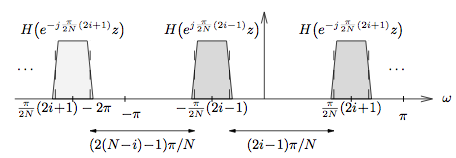Illustration of vanishing terms in [link] (lower equation). Next we concern ourselves with the requirements on a 0 and c 0 . Assuming [link] is satisfied, we know that inner aliasing in ${U}_{i}\left(z\right)$ cancels outer aliasing in ${U}_{i-1}\left(z\right)$ for $1\le i\le N-1$ . Hence, from [link] (fourth equation) and [link] (lower equation),
$\begin{array}{ccc}\hfill U\left(z\right)& =& \sum _{i=0}^{N-1}{U}_{i}\left(z\right)\hfill \\ & =& \frac{1}{N}\sum _{i=0}^{N-1}{K}_{i}\left(z\right){H}_{i}\left(z\right)X\left(z\right)\hfill \\ & =& \frac{1}{N}\sum _{i=0}^{N-1}\left[{c}_{i},H,\left({e}^{-j\frac{\pi }{2N}\left(2i+1\right)},z\right),+,{c}_{i}^{*},H,\left({e}^{j\frac{\pi }{2N}\left(2i+1\right)},z\right)\right]\hfill \\ & & \phantom{\rule{1.em}{0ex}}·\left[{a}_{i},H,\left({e}^{-j\frac{\pi }{2N}\left(2i+1\right)},z\right),+,{a}_{i}^{*},H,\left({e}^{j\frac{\pi }{2N}\left(2i+1\right)},z\right)\right]X\left(z\right)\hfill \end{array}$
Noting that the passbands of $H\left({e}^{-j\frac{\pi }{2N}\left(2i+1\right)},z\right)$ and $H\left({e}^{j\frac{\pi }{2N}\left(2i+1\right)},z\right)$ do not overlap for $1\le i\le N-2$ , we have
$\begin{array}{ccc}\hfill U\left(z\right)& =& \frac{1}{N}\left[\left({a}_{0}{c}_{0}^{*}+{a}_{0}^{*}{c}_{0}\right)H\left({e}^{-j\frac{\pi }{2N}},z\right)H\left({e}^{j\frac{\pi }{2N}},z\right)\hfill \\ & & \phantom{\rule{1.em}{0ex}}+\left({a}_{N-1}{c}_{N-1}^{*}+{a}_{N-1}^{*}{c}_{N-1}\right)H\left({e}^{-j\frac{\pi }{2N}\left(2N-1\right)},z\right)H\left({e}^{j\frac{\pi }{2N}\left(2N-1\right)},z\right)\hfill \\ & & \phantom{\rule{1.em}{0ex}}+\sum _{i=0}^{N-1}\left({a}_{i},{c}_{i},{H}^{2},\left({e}^{-j\frac{\pi }{2N}\left(2i+1\right)},z\right),+,{a}_{i}^{*},{c}_{i}^{*},{H}^{2},\left({e}^{j\frac{\pi }{2N}\left(2i+1\right)},z\right)\right)\right]X\left(z\right).\hfill \end{array}$
The first two terms in [link] (third equation) represent aliasing components that prevent flat overall response at $\omega =0$ and $\omega =\pi$ , respectively. These aliasing terms vanish when
$\begin{array}{c}\hfill \begin{array}{|c|}\hline \begin{array}{ccc}\hfill {a}_{0}{c}_{0}^{*}& =& -{a}_{0}^{*}{c}_{0}\hfill \\ \hfill {a}_{N-1}{c}_{N-1}^{*}& =& -{a}_{N-1}^{*}{c}_{N-1}\hfill \end{array}\\ \hline\end{array}\end{array}$
What remains is
$\begin{array}{ccc}\hfill U\left(z\right)& =& \frac{1}{N}\sum _{i=0}^{N-1}\left({a}_{i},{c}_{i},{H}^{2},\left({e}^{-j\frac{\pi }{2N}\left(2i+1\right)},z\right),+,{a}_{i}^{*},{c}_{i}^{*},{H}^{2},\left({e}^{j\frac{\pi }{2N}\left(2i+1\right)},z\right)\right)X\left(z\right).\hfill \end{array}$
• Phase Distortion: Perfect reconstruction requires that the analysis/synthesis system hasno phase distortion. To guarantee the absence of phase distortion, we require that thecomposite system
$\begin{array}{c}\hfill Q\left(z\right)\phantom{\rule{3.33333pt}{0ex}}:=\phantom{\rule{3.33333pt}{0ex}}\frac{U\left(z\right)}{X\left(z\right)}\phantom{\rule{3.33333pt}{0ex}}=\phantom{\rule{3.33333pt}{0ex}}\frac{1}{N}\sum _{i=0}^{N-1}{a}_{i}{c}_{i}{H}^{2}\left({e}^{-j\frac{\pi }{2N}\left(2i+1\right)},z\right)+{a}_{i}^{*}{c}_{i}^{*}{H}^{2}\left({e}^{j\frac{\pi }{2N}\left(2i+1\right)},z\right)\end{array}$
has a linear phase response. (Recall that a linear phase response is equivalent to a pure delayin the time domain.) This linear-phase constraint will provide the final condition used tospecify the constants $\left\{{a}_{i}\right\}$ and $\left\{{c}_{i}\right\}$ . We start by examining the impulse response of $Q\left(z\right)$ . Using a technique analogous to [link] (fifth equation), we can write
$\begin{array}{c}\hfill Q\left(z\right)\phantom{\rule{3.33333pt}{0ex}}=\phantom{\rule{3.33333pt}{0ex}}\frac{2}{N}\sum _{n=0}^{2M-2}\left(\sum _{i=0}^{N-1},\mathrm{Re},\left({a}_{i}{c}_{i}\right),cos,\left(\pi ,\frac{2i\phantom{\rule{-0.166667em}{0ex}}+\phantom{\rule{-0.166667em}{0ex}}1}{2N},n\right),-,\mathrm{Im},\left({a}_{i}{c}_{i}\right),sin,\left(\pi ,\frac{2i\phantom{\rule{-0.166667em}{0ex}}+\phantom{\rule{-0.166667em}{0ex}}1}{2N},n\right)\right)\left(\sum _{k},{h}_{k},{h}_{n-k}\right){z}^{-n}\end{array}$
Above, we have used the property that multiplication in the z -domain implies convolution in the time domain.For $Q\left(z\right)$ to be linear phase, it's impulse response must be symmetric. Let us assume that the prototype filter $H\left(z\right)$ is linear phase, so that $\left\{{h}_{n}\right\}$ is symmetric. Thus ${\sum }_{k}{h}_{m}{h}_{n-k}$ is symmetric about $n=M-1$ , and thus for linear phase $Q\left(z\right)$ , we require that the quantity
$\begin{array}{c}\hfill \sum _{i=0}^{N-1}\mathrm{Re}\left({a}_{i}{c}_{i}\right)cos\left(\pi ,\frac{2i\phantom{\rule{-0.166667em}{0ex}}+\phantom{\rule{-0.166667em}{0ex}}1}{2N},n\right)-\mathrm{Im}\left({a}_{i}{c}_{i}\right)sin\left(\pi ,\frac{2i\phantom{\rule{-0.166667em}{0ex}}+\phantom{\rule{-0.166667em}{0ex}}1}{2N},n\right)\end{array}$
is symmetric about $n=M-1$ , i.e.,
$\begin{array}{c}\hfill \sum _{i=0}^{N-1}\mathrm{Re}\left({a}_{i}{c}_{i}\right)cos\left(\pi ,\frac{2i\phantom{\rule{-0.166667em}{0ex}}+\phantom{\rule{-0.166667em}{0ex}}1}{2N},\left(M-1+n\right)\right)-\mathrm{Im}\left({a}_{i}{c}_{i}\right)sin\left(\pi ,\frac{2i\phantom{\rule{-0.166667em}{0ex}}+\phantom{\rule{-0.166667em}{0ex}}1}{2N},\left(M-1+n\right)\right)\\ =\sum _{i=0}^{N-1}\mathrm{Re}\left({a}_{i}{c}_{i}\right)cos\left(\pi ,\frac{2i\phantom{\rule{-0.166667em}{0ex}}+\phantom{\rule{-0.166667em}{0ex}}1}{2N},\left(M-1-n\right)\right)-\mathrm{Im}\left({a}_{i}{c}_{i}\right)sin\left(\pi ,\frac{2i\phantom{\rule{-0.166667em}{0ex}}+\phantom{\rule{-0.166667em}{0ex}}1}{2N},\left(M-1-n\right)\right)\end{array}$
for $n=0,\cdots ,M-1$ . Using trigonometric identities, it can be shown that the condition aboveis equivalent to
$\begin{array}{ccc}\hfill 0& =& \sum _{i=0}^{N-1}sin\left(\pi ,\frac{2i\phantom{\rule{-0.166667em}{0ex}}+\phantom{\rule{-0.166667em}{0ex}}1}{2N},n\right)\left[\mathrm{Re},\left({a}_{i}{c}_{i}\right),sin,\left(\pi ,\frac{2i\phantom{\rule{-0.166667em}{0ex}}+\phantom{\rule{-0.166667em}{0ex}}1}{2N},\left(M-1\right)\right),+,\mathrm{Im},\left({a}_{i}{c}_{i}\right),cos,\left(\pi ,\frac{2i\phantom{\rule{-0.166667em}{0ex}}+\phantom{\rule{-0.166667em}{0ex}}1}{2N},\left(M-1\right)\right)\right],\hfill \end{array}$
which is satisfied when
$\begin{array}{ccc}\hfill \frac{\mathrm{Im}\left({a}_{i}{c}_{i}\right)}{\mathrm{Re}\left({a}_{i}{c}_{i}\right)}& =& -\frac{sin\left(\pi ,\frac{2i\phantom{\rule{-0.166667em}{0ex}}+\phantom{\rule{-0.166667em}{0ex}}1}{2N},\left(M-1\right)\right)}{cos\left(\pi ,\frac{2i\phantom{\rule{-0.166667em}{0ex}}+\phantom{\rule{-0.166667em}{0ex}}1}{2N},\left(M-1\right)\right)}\phantom{\rule{3.33333pt}{0ex}}=\phantom{\rule{3.33333pt}{0ex}}tan\left(-,\pi ,\frac{2i\phantom{\rule{-0.166667em}{0ex}}+\phantom{\rule{-0.166667em}{0ex}}1}{2N},\left(M-1\right)\right).\hfill \end{array}$
Restricting $|{a}_{i}|=|{c}_{i}|=1$ , the previous equation requires that
$\begin{array}{c}\hfill \begin{array}{|c|}\hline {a}_{i}{c}_{i}\phantom{\rule{3.33333pt}{0ex}}=\phantom{\rule{3.33333pt}{0ex}}{e}^{-j\pi \frac{2i+1}{2N}\left(M-1\right)}\\ \hline\end{array}.\end{array}$
It can be easily verified that the following $\left\{{a}_{i}\right\}$ and $\left\{{c}_{i}\right\}$ satisfy conditions [link] , [link] , and [link] :
$\begin{array}{c}\hfill \begin{array}{|c|}\hline \begin{array}{ccc}\hfill {a}_{i}& =& {e}^{-j\pi \frac{M+N-1}{4N}\left(2i+1\right)}\hfill \\ \hfill {c}_{i}& =& {e}^{-j\pi \frac{M-N-1}{4N}\left(2i+1\right)}.\hfill \end{array}\\ \hline\end{array}\end{array}$
Plugging these into the expression for ${H}_{i}\left(z\right)$ we find that
$\begin{array}{ccc}\hfill {H}_{i}\left(z\right)& =& {a}_{i}H\left({e}^{-j\pi \frac{2i+1}{2N}},z\right)+{a}_{i}^{*}H\left({e}^{j\pi \frac{2i+1}{2N}},z\right)\hfill \\ & =& \sum _{n=0}^{M-1}\left({a}_{i},{e}^{j\pi \frac{2i+1}{2N}n},+,{a}_{i}^{*},{e}^{-j\pi \frac{2i+1}{2N}n}\right){h}_{n}{z}^{-n}\hfill \\ & =& \sum _{n=0}^{M-1}\left({e}^{j\pi \frac{2i+1}{2N}\left(n-\frac{M+N-1}{2}\right)},+,{e}^{-j\pi \frac{2i+1}{2N}\left(n-\frac{M+N-1}{2}\right)}\right){h}_{n}{z}^{-n}\hfill \\ & =& \sum _{n=0}^{M-1}\underset{\text{impulse}\phantom{\rule{4.pt}{0ex}}\text{response}\phantom{\rule{4.pt}{0ex}}\text{of}\phantom{\rule{4.pt}{0ex}}{H}_{i}\left(z\right)}{\underbrace{\phantom{\rule{0.166667em}{0ex}}\begin{array}{|c|}\hline 2cos\left(\pi ,\frac{2i+1}{2N},\left(n,-,\frac{M+N-1}{2}\right)\right){h}_{n}\\ \hline\end{array}\phantom{\rule{0.166667em}{0ex}}}}{z}^{-n}.\hfill \end{array}$
Repeating this procedure for ${K}_{i}\left(z\right)$ yields
$\begin{array}{ccc}\hfill {K}_{i}\left(z\right)& =& \sum _{n=0}^{M-1}\underset{\text{impulse}\phantom{\rule{4.pt}{0ex}}\text{response}\phantom{\rule{4.pt}{0ex}}\text{of}\phantom{\rule{4.pt}{0ex}}{K}_{i}\left(z\right)}{\underbrace{\phantom{\rule{0.166667em}{0ex}}\begin{array}{|c|}\hline 2cos\left(\pi ,\frac{2i+1}{2N},\left(n,-,\frac{M-N-1}{2}\right)\right){h}_{n}\\ \hline\end{array}\phantom{\rule{0.166667em}{0ex}}}}{z}^{-n}.\hfill \end{array}$
At this point we make a few comments on the design of the lowpass prototype $H\left(z\right)$ . The perfect $H\left(z\right)$ would be an ideal linear-phase lowpass filter with cutoff at $\omega =\pi /2N$ , as illustrated in [link] . Such a filter would perfectly separate the subbands as well as yieldflat composite magnitude response, as per [link] . Unfortunately, however, this perfect filter is not realizable with afinite number of filter coefficients. So, what we really want is a finite-length FIR filter having goodfrequency selectivity, nearly-flat composite response, and linear phase. The length-512 prototype filter specified in the MPEG standards issuch a filter, as evidenced by the responses in [link] . Unfortunately, the standards do not describe how this filter was designed,and a thorough discussion of multirate filter design is outside the scope of this course. For more on prototype filter design, we point the interested reader to page 358 of Vaidyanathan or Crochiere&Rabiner.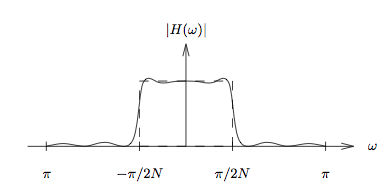Ideal (dashed) and typical (solid) prototype-filter magnitude responses for the cosine-modulated filterbank. Note bandwidth relative to [link] .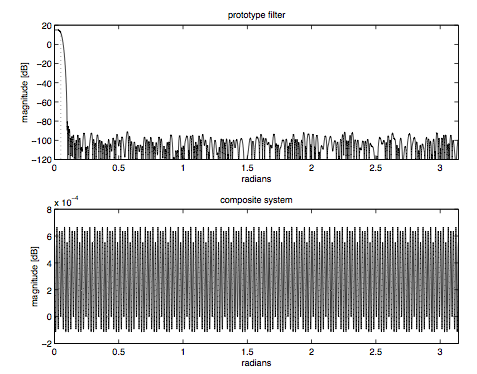Magnitude response of | H ( ω ) | of MPEG prototype filter and the resulting composite response | Q ( ω ) | , where N = 32 and M = 16 N = 512 . To conclude, [link] (fourth equation) and [link] give impulse response expressions for a set of real-valued filters that comprise a near-perfectlyreconstructing filterbank (under suitable selection of $\left\{{h}_{i}\right\}$ ). This is commonly referred to The MPEG standards refer to this filterbank as a “polyphase quadrature” filterbank (PQF), the name given to the technique byan early technical paper: Rothweiler ICASSP 83 as a “cosine-modulated filterbank” because all filters are based on cosine modulations of areal-valued linear-phase lowpass prototype $H\left(z\right)$ . The near-perfect reconstruction property follows from the frequency-domaincancellation of adjacent-spectrum aliasing and the lack of phase distortion.
It should be noted that our derivation of the cosine modulated filterbank is similar to that in Rothweiler ICASSP 83 except for the treatmentsof phase distortion. See Chapter 8 of Vaidyanathan for a more comprehensiveview of cosine-modulated filterbanks.
• Polyphase Implementations: Recall the uniformly modulated filterbank in Figure 4 from "Uniformly-Modulated Filterbanks" , whose combined modulator-filter coefficients can be constructed using products of the terms ${h}_{n}$ and ${e}^{j\frac{\pi }{N}in}$ . Figure 5 from "Uniformly-Modulated Filterbanks" shows a computationally-efficient polyphase/DFT implementation of the analysis filter which requires only M multiplies and one N -dimensional DFT computation for calculation of N subband outputs.We might wonder: Is there a similar polyphase/fast-transform implementation of the cosine-modulated filterbank derived inthis section? From [link] (fourth equation), we see that the impulse responses of $\left\{{H}_{i}\left(z\right)\right\}$ are products of the terms ${h}_{n}$ and $cos\left(\pi ,\frac{2i+1}{2N},\left(n,-,\frac{M+N-1}{2}\right)\right)$ for $n=0,\cdots ,M-1$ . Note that the inverse-DCT matrix C n t can be specified via components with form similar to the cosine term in [link] (fourth equation):
$\begin{array}{ccc}\hfill {\left[{\mathbf{C}}_{N}^{t}\right]}_{i,n}& =& \sqrt{\frac{2}{N}}\phantom{\rule{0.166667em}{0ex}}{\alpha }_{n}cos\left(\pi ,\frac{\left(2i+1\right)}{2N},n\right);\phantom{\rule{1.em}{0ex}}i,n=0\cdots N\phantom{\rule{-0.166667em}{0ex}}-\phantom{\rule{-0.166667em}{0ex}}1.\hfill \\ & & \text{for}\phantom{\rule{3.33333pt}{0ex}}\phantom{\rule{3.33333pt}{0ex}}{\alpha }_{0}=1/\sqrt{2},\phantom{\rule{3.33333pt}{0ex}}\phantom{\rule{3.33333pt}{0ex}}{\alpha }_{n\ne 0}=1.\hfill \end{array}$
Thus it may not be surprising that there exist polyphase/DCT implementations of the cosine-modulated filterbank.Indeed, one such implementation is specified in the MPEG-2 audio compression standard (see ISO/IEC 13818-3).This particular implementation is the focus of the next section.

## Mpeg filterbank implementation

• Since MPEG audio compression standards are so well-known and widespread, a detailed look at the MPEG filterbank implementation is warranted.The cosine-modulated, or polyphase-quadrature filterbank described in the previous section is used in MPEG Layers 1-3.(The MPEG hierarchy will be described in a later chapter.) This section discusses the specific implementation suggested by theMPEG-2 standard (see ISO/IEC 13818-3).
• The MPEG standard specifies 512 prototype filter coefficients, the first of which is zero.To adapt the MPEG filter to our cosine-modulated-filterbank framework, we append a zero-valued 513 th coefficient so that the resulting MPEG prototype filter becomes symmetric and hence linear phase.Since the standard specifies $N=32$ frequency bands, we have
$M\phantom{\rule{3.33333pt}{0ex}}=\phantom{\rule{3.33333pt}{0ex}}513\phantom{\rule{3.33333pt}{0ex}}=\phantom{\rule{3.33333pt}{0ex}}16N+1.$
Plugging this value of M into the filter expressions [link] (fourth equation) and [link] , the $2\pi$ -periodicity of the cosine implies that they may be rewritten as follows.
$\begin{array}{ccc}\hfill {H}_{i}\left(z\right)& =& \sum _{n=0}^{16N-1}\underset{\text{impulse}\phantom{\rule{4.pt}{0ex}}\text{response}\phantom{\rule{4.pt}{0ex}}\text{of}\phantom{\rule{4.pt}{0ex}}{H}_{i}\left(z\right)}{\underbrace{\phantom{\rule{0.166667em}{0ex}}2cos\left(\pi ,\frac{2i+1}{2N},\left(n,-,\frac{N}{2}\right)\right){h}_{n}\phantom{\rule{0.166667em}{0ex}}}}{z}^{-n}\hfill \\ \hfill {K}_{i}\left(z\right)& =& \sum _{n=0}^{16N-1}\underset{\text{impulse}\phantom{\rule{4.pt}{0ex}}\text{response}\phantom{\rule{4.pt}{0ex}}\text{of}\phantom{\rule{4.pt}{0ex}}{K}_{i}\left(z\right)}{\underbrace{\phantom{\rule{0.166667em}{0ex}}2cos\left(\pi ,\frac{2i+1}{2N},\left(n,+,\frac{N}{2}\right)\right){h}_{n}\phantom{\rule{0.166667em}{0ex}}}}{z}^{-n}.\hfill \end{array}$
• Encoding: Here we derive the encoder filterbank implementation suggested in theMPEG-2 standard (see ISO/IEC 13818-3). Using ${x}_{i}\left(n\right)$ to denote the output of the ${i}^{th}$ analysis filter, we have
${x}_{i}\left(n\right)\phantom{\rule{3.33333pt}{0ex}}=\phantom{\rule{3.33333pt}{0ex}}\sum _{k=0}^{16N-1}\left[2cos\left(\pi ,\frac{2i+1}{2N},\left(,k,-,\frac{N}{2}\right)\right){h}_{k}\right]x\left(n-k\right).$
The relationship between ${x}_{i}\left(n\right)$ and its downsampled version ${s}_{i}\left(m\right)$ is given by
${s}_{i}\left(m\right)={x}_{i}\left(mN\right),$
so that the downsampled analysis output ${s}_{i}\left(m\right)$ can be written as
$\begin{array}{ccc}\hfill {s}_{i}\left(m\right)& =& \sum _{n=0}^{16N-1}\left[2cos\left(\pi ,\frac{2i+1}{2N},\left(,n,-,\frac{N}{2}\right)\right){h}_{n}\right]x\left(mN-n\right).\hfill \end{array}$
Using the substitution $n=kN+\ell$ for $0\le \ell \le N\phantom{\rule{-0.166667em}{0ex}}-\phantom{\rule{-0.166667em}{0ex}}1$ ,
$\begin{array}{ccc}\hfill {s}_{i}\left(m\right)& =& 2\sum _{k=0}^{15}\sum _{\ell =0}^{N-1}\underset{\begin{array}{c}\text{repeats}\phantom{\rule{4.pt}{0ex}}\text{every}\phantom{\rule{4.pt}{0ex}}4\phantom{\rule{4.pt}{0ex}}\text{increments}\phantom{\rule{4.pt}{0ex}}\text{of}\phantom{\rule{4.pt}{0ex}}k\\ \text{sign}\phantom{\rule{4.pt}{0ex}}\text{changes}\phantom{\rule{4.pt}{0ex}}\text{every}\phantom{\rule{4.pt}{0ex}}2\phantom{\rule{4.pt}{0ex}}\text{increments}\phantom{\rule{4.pt}{0ex}}\text{of}\phantom{\rule{4.pt}{0ex}}k\end{array}}{\underbrace{cos\left(\pi ,\frac{2i+1}{2N},\left(k,N,+,\ell ,-,\frac{N}{2}\right)\right)}}{h}_{kN+\ell }\phantom{\rule{0.277778em}{0ex}}x\left(\left(,m,-,k,\right),N,-,\ell \right)\hfill \\ & =& \sum _{k=0}^{15}\sum _{\ell =0}^{N-1}\underset{\text{repeats}\phantom{\rule{4.pt}{0ex}}\text{every}\phantom{\rule{4.pt}{0ex}}2\phantom{\rule{4.pt}{0ex}}\text{increments}\phantom{\rule{4.pt}{0ex}}\text{of}\phantom{\rule{4.pt}{0ex}}k}{\underbrace{cos\left(\pi ,\frac{2i+1}{2N},\left({⟨k⟩}_{2},N,+,\ell ,-,\frac{N}{2}\right)\right)}}\phantom{\rule{0.277778em}{0ex}}\underset{\text{analysis}\phantom{\rule{4.pt}{0ex}}\text{window}}{\underbrace{2\phantom{\rule{0.166667em}{0ex}}{\left(-1\right)}^{⌊k/2⌋}{h}_{kN+\ell }}}\phantom{\rule{0.277778em}{0ex}}x\left(\left(,m,-,k,\right),N,-,\ell \right)\hfill \end{array}$
• Decoding: Here we derive the dencoder filterbank implementation suggested in theMPEG-2 standard (see ISO/IEC 13818-3). Using ${y}_{i}\left(n\right)$ to denote the output of the ${i}^{th}$ upsampler,
$\begin{array}{ccc}\hfill {u}_{i}\left(n\right)& =& \sum _{k=0}^{16N-1}\left[2cos\left(\pi ,\frac{2i+1}{2N},\left(k,+,\frac{N}{2}\right)\right){h}_{k}\right]{y}_{i}\left(n-k\right).\hfill \end{array}$
The input to the upsampler ${s}_{i}\left(m\right)$ is related to the output ${y}_{i}\left(n\right)$ by
${y}_{i}\left(n\right)\phantom{\rule{3.33333pt}{0ex}}=\phantom{\rule{3.33333pt}{0ex}}\left\{\begin{array}{cc}{s}_{i}\left(n/N\right)\hfill & \text{when}\phantom{\rule{3.33333pt}{0ex}}n/N\in \mathbb{Z}\hfill \\ 0\hfill & \text{else},\hfill \end{array}\right)$
so that
$\begin{array}{ccc}\hfill {u}_{i}\left(n\right)& =& \sum _{\left\{k:\frac{n-k}{N}\in \mathbb{Z}\right\}}\left[2cos\left(\pi ,\frac{2i+1}{2N},\left(k,+,\frac{N}{2}\right)\right){h}_{k}\right]{s}_{i}\left(\frac{n-k}{N}\right).\hfill \end{array}$
Lets write $n=mN+\ell$ for $0\le \ell \le N\phantom{\rule{-0.166667em}{0ex}}-\phantom{\rule{-0.166667em}{0ex}}1$ and $k=pN+q$ for $0\le q\le N\phantom{\rule{-0.166667em}{0ex}}-\phantom{\rule{-0.166667em}{0ex}}1$ . Then due to the restricted ranges of and q ,
$\frac{n-k}{N}\phantom{\rule{3.33333pt}{0ex}}=\phantom{\rule{3.33333pt}{0ex}}m-p+\frac{\ell -q}{N}\phantom{\rule{3.33333pt}{0ex}}\in \phantom{\rule{3.33333pt}{0ex}}\mathbb{Z}\phantom{\rule{1.em}{0ex}}⇒\phantom{\rule{1.em}{0ex}}\ell \phantom{\rule{3.33333pt}{0ex}}=\phantom{\rule{3.33333pt}{0ex}}q.$
Using these substitutions in the previous equation for ${u}_{i}\left(n\right)$ ,
$\begin{array}{ccc}\hfill {u}_{i}\left(mN+\ell \right)& =& 2\sum _{p=0}^{15}cos\left(\pi ,\frac{2i+1}{2N},\left(p,N,+,\ell ,+,\frac{N}{2}\right)\right){h}_{pN+\ell }\phantom{\rule{0.222222em}{0ex}}{s}_{i}\left(m-p\right).\hfill \end{array}$
Summing ${u}_{i}\left(mN+\ell \right)$ over i to create $u\left(mN+\ell \right)$ ,
$\begin{array}{ccc}\hfill u\left(mN+\ell \right)& =& 2\sum _{i=0}^{N-1}\sum _{p=0}^{15}\underset{\begin{array}{c}\text{repeats}\phantom{\rule{4.pt}{0ex}}\text{every}\phantom{\rule{4.pt}{0ex}}4\phantom{\rule{4.pt}{0ex}}\text{increments}\phantom{\rule{4.pt}{0ex}}\text{of}\phantom{\rule{4.pt}{0ex}}p\\ \text{sign}\phantom{\rule{4.pt}{0ex}}\text{changes}\phantom{\rule{4.pt}{0ex}}\text{every}\phantom{\rule{4.pt}{0ex}}2\phantom{\rule{4.pt}{0ex}}\text{increments}\phantom{\rule{4.pt}{0ex}}\text{of}\phantom{\rule{4.pt}{0ex}}p\end{array}}{\underbrace{cos\left(\pi ,\frac{2i+1}{2N},\left(p,N,+,\ell ,+,\frac{N}{2}\right)\right)\right)}}{h}_{pN+\ell }\phantom{\rule{0.222222em}{0ex}}{s}_{i}\left(m-p\right)\hfill \\ & =& \sum _{p=0}^{15}\underset{\text{synthesis}\phantom{\rule{4.pt}{0ex}}\text{window}}{\underbrace{2\phantom{\rule{0.166667em}{0ex}}{\left(-1\right)}^{⌊p/2⌋}{h}_{pN+\ell }}}\phantom{\rule{5.69054pt}{0ex}}\sum _{i=0}^{N-1}\phantom{\rule{-8.53581pt}{0ex}}\underset{=\left\{\begin{array}{cc}cos\left(\pi ,\frac{2i+1}{2N},\left(\ell ,+,\frac{N}{2}\right)\right)\hfill & p\phantom{\rule{4.pt}{0ex}}\text{even}\hfill \\ cos\left(\pi ,\frac{2i+1}{2N},\left(\ell ,+,N,+,\frac{N}{2}\right)\right)\hfill & p\phantom{\rule{4.pt}{0ex}}\text{odd}\hfill \end{array}\right)}{\underbrace{cos\left(\pi ,\frac{2i+1}{2N},\left({⟨p⟩}_{2},N,+,\ell ,+,\frac{N}{2}\right)\right)\right)}}{s}_{i}\left(m-p\right)\hfill \end{array}$
If we define
${v}_{j}\left(m\right)\phantom{\rule{3.33333pt}{0ex}}=\phantom{\rule{3.33333pt}{0ex}}\sum _{i=0}^{N-1}cos\left(\pi ,\frac{2i+1}{2N},\left(j,+,\frac{N}{2}\right)\right){s}_{i}\left(m\right)\phantom{\rule{1.em}{0ex}}\text{for}\phantom{\rule{1.em}{0ex}}0\le j\le 2N-1,$
(note the range of $j\phantom{\rule{0.166667em}{0ex}}$ !) then we can rewrite
$\begin{array}{c}\hfill u\left(mN+\ell \right)\phantom{\rule{3.33333pt}{0ex}}=\sum _{p=0,2,\cdots ,14}{\left(-1\right)}^{⌊p/2⌋}{h}_{pN+\ell }\phantom{\rule{0.222222em}{0ex}}{v}_{\ell }\left(m-p\right)\phantom{\rule{8.53581pt}{0ex}}+\phantom{\rule{-2.84526pt}{0ex}}\sum _{p=1,3,\cdots ,15}{\left(-1\right)}^{⌊p/2⌋}{h}_{pN+\ell }\phantom{\rule{0.222222em}{0ex}}{v}_{\ell +N}\left(m-p\right).\end{array}$
[link] illustrates the construction of $u\left(mN+\ell \right)$ using the notation
$\mathbf{v}\left(m\right)\phantom{\rule{3.33333pt}{0ex}}=\left(\begin{array}{ccc}{v}_{0}\left(m\right)& \cdots & {v}_{2N-1}\left(m\right)\end{array}\right).$
• DCT Implementation of Cosine Matrixing: As seen in [link] and [link] , the filterbank implementations suggested by the MPEG standard requirea cosine matrix operation that, if implemented using straightforward arithmetic, requires $32×64=2048$ multiply/adds at both the encoder and decoder.Note, however, that the cosine transformations in [link] and [link] do bear a great deal of similarity to the DCT:
$\begin{array}{ccc}\hfill {y}_{k}& =& \sqrt{\frac{2}{N}}\phantom{\rule{0.166667em}{0ex}}{\alpha }_{k}\sum _{n=0}^{N-1}{x}_{n}cos\left(\pi ,\frac{2n+1}{2N},k\right);\phantom{\rule{1.em}{0ex}}k=0\cdots N\phantom{\rule{-0.166667em}{0ex}}-\phantom{\rule{-0.166667em}{0ex}}1,\hfill \\ & & \text{for}\phantom{\rule{3.33333pt}{0ex}}\phantom{\rule{3.33333pt}{0ex}}{\alpha }_{0}=1/\sqrt{2},\phantom{\rule{3.33333pt}{0ex}}\phantom{\rule{3.33333pt}{0ex}}{\alpha }_{k\ne 0}=1,\hfill \\ \hfill {x}_{n}& =& \sqrt{\frac{2}{N}}\sum _{k=0}^{N-1}{\alpha }_{k}\phantom{\rule{0.166667em}{0ex}}{y}_{k}cos\left(\pi ,\frac{2n+1}{2N},k\right);\phantom{\rule{1.em}{0ex}}n=0\cdots N\phantom{\rule{-0.166667em}{0ex}}-\phantom{\rule{-0.166667em}{0ex}}1,\hfill \end{array}$
which we know has a fast algorithm: Lee's $32×32$ fast-DCT, for example, requires only 80 multiplications and 209 additions (see B.G.Lee TASSP Dec 84).So how do we implement the matrix operation using the fast-DCT? A technique has been described clearly in Konstantinides SPL 1994,the results of which are summarized below. At the encoder, the matrix operation can be written
$\begin{array}{ccc}\hfill {s}_{i}\left(m\right)& =& \sum _{j=0}^{2N-1}cos\left(\pi ,\frac{2i+1}{2N},\left(j,-,\frac{N}{2}\right)\right){w}_{j}\left(m\right)\phantom{\rule{1.em}{0ex}}\text{for}\phantom{\rule{1.em}{0ex}}i=0,\cdots ,N-1,\hfill \end{array}$
where $\left\{{w}_{0}\left(m\right),\cdots ,{w}_{2N-1}\left(m\right)$ is created from $\left\{x\left(m\right),\cdots ,x\left(m-16N+1\right)\right\}$ by windowing, shifting, and adding. (See [link] .) We can write
$\begin{array}{ccc}\hfill {s}_{i}\left(m\right)& =& \sum _{j=0}^{N-1}cos\left(\pi ,\frac{2i+1}{2N},j\right){\overline{w}}_{j}\left(m\right);\phantom{\rule{1.em}{0ex}}i=0,\cdots ,N-1,\hfill \end{array}$
where, for $N=32$ , $\left\{{\overline{w}}_{j}\left(m\right)\right\}$ is the following manipulation of $\left\{{w}_{j}\left(m\right)\right\}$ :
$\begin{array}{c}\hfill {\overline{w}}_{j}\left(m\right)\phantom{\rule{3.33333pt}{0ex}}:=\phantom{\rule{3.33333pt}{0ex}}\left\{\begin{array}{cc}{w}_{16}\left(m\right)\hfill & j=0\hfill \\ {w}_{16+j}\left(m\right)+{w}_{16-j}\left(m\right)\hfill & j=1,2,\cdots ,16\hfill \\ {w}_{16+j}\left(m\right)-{w}_{80-j}\left(m\right)\hfill & j=17,18,\cdots ,31.\hfill \end{array}\right)\end{array}$
Compare [link] to the inverse DCT in [link] (lower equation). At the decoder, the matrix operation can be written
$\begin{array}{ccc}\hfill {v}_{j}\left(m\right)& =& \sum _{i=0}^{N-1}cos\left(\pi ,\frac{2i+1}{2N},\left(j,+,\frac{N}{2}\right)\right){s}_{i}\left(m\right)\phantom{\rule{1.em}{0ex}}\text{for}\phantom{\rule{1.em}{0ex}}j=0,\cdots ,2N-1,\hfill \end{array}$
where $\left\{{v}_{0}\left(m\right),\cdots ,{v}_{2N-1}\left(m\right)\right\}$ are windowed, shifted, and added to compute $\left\{u\left(m\right)\right\}$ . (See [link] .) It is shown in Konstantinides SPL 1994 that, for $N=32$ , $\left\{{v}_{j}\left(m\right)\right\}$ can be calculated by first computing $\left\{{\overline{v}}_{j}\left(m\right)\right\}$ :
$\begin{array}{ccc}\hfill {\overline{v}}_{j}\left(m\right)& =& \sum _{i=0}^{N-1}cos\left(\pi ,\frac{2i+1}{2N},j\right){s}_{i}\left(m\right);\phantom{\rule{1.em}{0ex}}j=0,\cdots ,N-1\hfill \end{array}$
and rearranging the outputs according to
$\begin{array}{c}\hfill {v}_{j}\left(m\right)\phantom{\rule{3.33333pt}{0ex}}:=\phantom{\rule{3.33333pt}{0ex}}\left\{\begin{array}{cc}{\overline{v}}_{j+16}\left(m\right)\hfill & j=0,1,\cdots ,15,\hfill \\ 0\hfill & j=16,\hfill \\ -{\overline{v}}_{48-j}\left(m\right)\hfill & j=17,18,\cdots ,47,\hfill \\ -{\overline{v}}_{j-48}\left(m\right)\hfill & j=48,49,\cdots ,63.\hfill \end{array}\right)\end{array}$

are nano particles real
yeah
Joseph
Hello, if I study Physics teacher in bachelor, can I study Nanotechnology in master?
no can't
Lohitha
where we get a research paper on Nano chemistry....?
nanopartical of organic/inorganic / physical chemistry , pdf / thesis / review
Ali
what are the products of Nano chemistry?
There are lots of products of nano chemistry... Like nano coatings.....carbon fiber.. And lots of others..
learn
Even nanotechnology is pretty much all about chemistry... Its the chemistry on quantum or atomic level
learn
da
no nanotechnology is also a part of physics and maths it requires angle formulas and some pressure regarding concepts
Bhagvanji
hey
Giriraj
Preparation and Applications of Nanomaterial for Drug Delivery
revolt
da
Application of nanotechnology in medicine
has a lot of application modern world
Kamaluddeen
yes
narayan
what is variations in raman spectra for nanomaterials
ya I also want to know the raman spectra
Bhagvanji
I only see partial conversation and what's the question here!
what about nanotechnology for water purification
please someone correct me if I'm wrong but I think one can use nanoparticles, specially silver nanoparticles for water treatment.
Damian
yes that's correct
Professor
I think
Professor
Nasa has use it in the 60's, copper as water purification in the moon travel.
Alexandre
nanocopper obvius
Alexandre
what is the stm
is there industrial application of fullrenes. What is the method to prepare fullrene on large scale.?
Rafiq
industrial application...? mmm I think on the medical side as drug carrier, but you should go deeper on your research, I may be wrong
Damian
How we are making nano material?
what is a peer
What is meant by 'nano scale'?
What is STMs full form?
LITNING
scanning tunneling microscope
Sahil
how nano science is used for hydrophobicity
Santosh
Do u think that Graphene and Fullrene fiber can be used to make Air Plane body structure the lightest and strongest. Rafiq
Rafiq
what is differents between GO and RGO?
Mahi
what is simplest way to understand the applications of nano robots used to detect the cancer affected cell of human body.? How this robot is carried to required site of body cell.? what will be the carrier material and how can be detected that correct delivery of drug is done Rafiq
Rafiq
if virus is killing to make ARTIFICIAL DNA OF GRAPHENE FOR KILLED THE VIRUS .THIS IS OUR ASSUMPTION
Anam
analytical skills graphene is prepared to kill any type viruses .
Anam
Any one who tell me about Preparation and application of Nanomaterial for drug Delivery
Hafiz
what is Nano technology ?
write examples of Nano molecule?
Bob
The nanotechnology is as new science, to scale nanometric
brayan
nanotechnology is the study, desing, synthesis, manipulation and application of materials and functional systems through control of matter at nanoscale
Damian
Is there any normative that regulates the use of silver nanoparticles?
what king of growth are you checking .?
Renato
Got questions? Join the online conversation and get instant answers!

#### Get Jobilize Job Search Mobile App in your pocket Now!By Brooke DelaneyBy OpenStaxBy OpenStaxBy Jessica CollettBy Mistry BhaveshBy Brooke DelaneyBy OpenStaxBy Madison ChristianBy Jordon HumphreysBy Rohini Ajay